Next: SLA_DCS2C - Spherical to Cartesian
Up: SUBPROGRAM SPECIFICATIONS
Previous: SLA_DCC2S - Cartesian to Spherical

## SLA_DCMPF - Interpret Linear Fit

ACTION:
Decompose an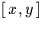linear fit into its constituent parameters: zero points, scales, nonperpendicularity and orientation.

CALL:
CALL sla_DCMPF (COEFFS,XZ,YZ,XS,YS,PERP,ORIENT)

GIVEN:

 D(6) COEFFS transformation coefficients (see note)

RETURNED:

 D x zero point XZ YZ D y zero point XS D x scale YS D y scale PERP D nonperpendicularity (radians) ORIENT D orientation (radians)

NOTES:
1.
The model relates two sets ofcoordinates as follows. Naming the six elements of COEFFS a,b,c,d,e & f, the model transforms coordinates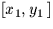into coordinates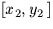as follows:
x2 = a + bx1 + cy1
y2 = d + ex1 + fy1
The sla_DCMPF routine decomposes this transformation into four steps:
(a)
Zero points: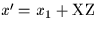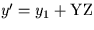(b)
Scales: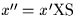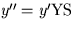(c)
Nonperpendicularity: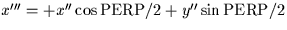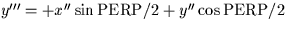(d)
Orientation: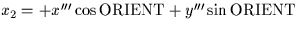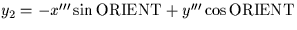2.